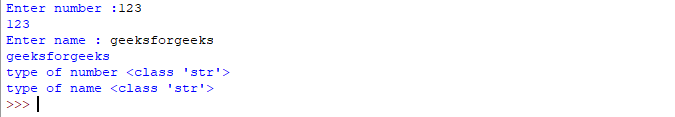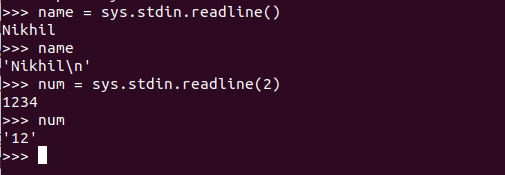# Difference between input() and sys.stdin.readline()

## Difference between input() and sys.stdin.readline()

Python is a widely used general-purpose language that can be used for many purposes. Taking input in any language is as important as breathing for humans. Python provides various methods for taking input. However, we all may get confused about how each method is different from one another. In this article, we will discuss about two such methods i.e `input()` and `sys.stdin.readline()`.

## Input()

This function first takes the input from the user and then evaluates the expression, which means Python automatically identifies whether the user entered a string or a number or list. If the input provided is not correct then either syntax error or exception is raised by Python.

How the input function works in Python :

• When `input()` function executes program flow will be stopped until the user has given input.
• The text or message display on the output screen to ask a user to enter input value is optional i.e. the prompt, will be printed on the screen is optional.
• Whatever you enter as input, input function converts it into a string. if you enter an integer value still input() function converts it into a string. You need to explicitly convert it into an integer in your code using typecasting.

Example:

 `# Program to check input  ` `# type in Python ` `   ` `num ``=` `input` `(``"Enter number :"``) ` `print``(num) ` `name1 ``=` `input``(``"Enter name : "``) ` `print``(name1) ` `   ` `# Printing type of input value ` `print` `(``"type of number"``, ``type``(num)) ` `print` `(``"type of name"``, ``type``(name1))`

Output:Stdin stands for standard input which is a stream from which the program reads its input data. This method is slightly different from the input() method as it also reads the escape character entered by the user. More this method also provides the parameter for the size i.e. how many characters it can read at a time.

Example:

 `# Python program to demonstrate` `# sys.stdin.readline()` ` ` ` ` `import` `sys` ` ` `name ``=` `sys.stdin.readline()` `print``(name)` ` ` `num ``=` `sys.stdin.readline(``2``)` `print``(num)`

Output:#### Difference between Input and sys.stdin.readline() function.

The input takes input from the user but does not read escape character. The readline() also takes input from the user but also reads the escape character.
It has a prompt that represents the default value before the user input. Readline has a parameter named size, Which is a non-negative number, it actually defines the bytes to be read.

Last Updated on March 1, 2022 by admin

## Get directory of current Python scriptGet directory of current Python script

Get directory of current Python script While working with file handling you might have noticed that files

## numpy.square() in Pythonnumpy.square() in Python

numpy.square() in Python numpy.square(arr, out = None, ufunc ‘square’) : This mathematical function helps user to

## Python – Draw Star Using Turtle GraphicsPython – Draw Star Using Turtle Graphics

Python – Draw Star Using Turtle Graphics In this article, we will learn how to

## Python – Largest, Smallest, Second Largest, Second Smallest in a ListPython – Largest, Smallest, Second Largest, Second Smallest in a List

Python | Largest, Smallest, Second Largest, Second Smallest in a List Since, unlike other programming

## Python program to input a comma separated stringPython program to input a comma separated string

Python program to input a comma separated string Given an input string that is comma-separated

## How to Plot Mean and Standard Deviation in Pandas?How to Plot Mean and Standard Deviation in Pandas?

How to Plot Mean and Standard Deviation in Pandas? Errorbar is the plotted chart that refers

## Python – pass multiple arguments to map functionPython – pass multiple arguments to map function

Python – pass multiple arguments to map function The map() function is a built-in function

## First Class functions in PythonFirst Class functions in Python

First Class functions in Python First class objects in a language are handled uniformly throughout. They